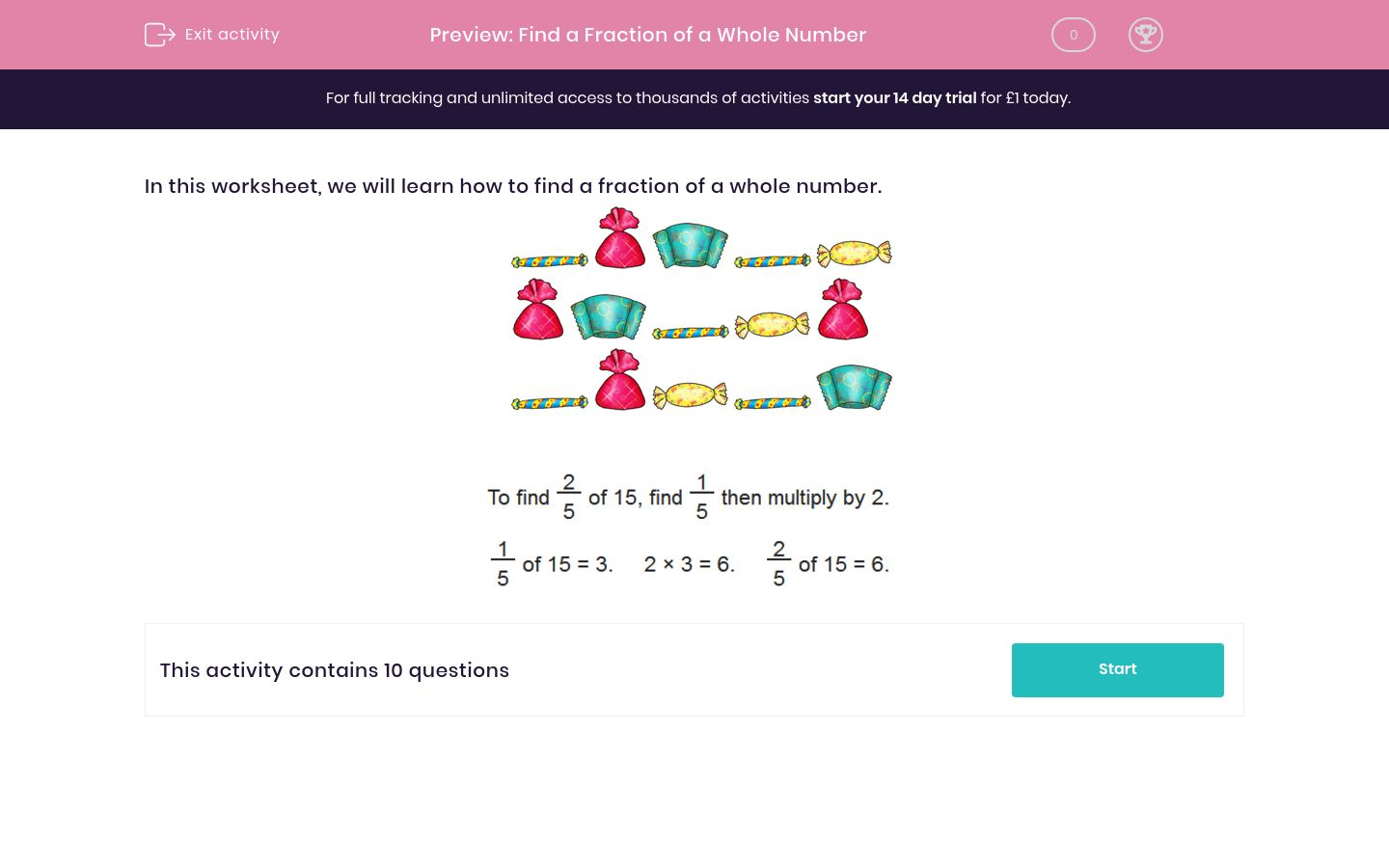# Find a Fraction of a Whole Number

In this worksheet, students evaluate a fraction of a whole number.Key stage:  KS 2

Curriculum topic:   Maths and Numerical Reasoning

Curriculum subtopic:   Fractions

Difficulty level:### QUESTION 1 of 10

In this worksheet, we will learn how to find a fraction of a whole number.---- OR ----